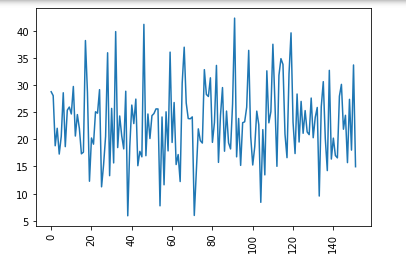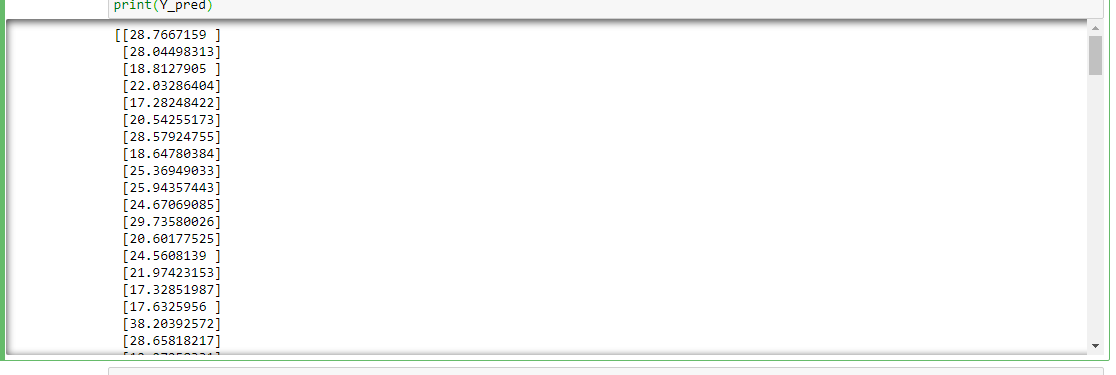Open in App
Not now

# sklearn.cross_decomposition.PLSRegression() function in Python

• Last Updated : 03 Jun, 2021

PLS regression is a Regression method that takes into account the latent structure in both datasets. Partial least squares regression performed well in MRI-based assessments for both single-label and multi-label learning reasons. PLSRegression acquires from PLS with mode=”A” and deflation_mode=”regression”. Additionally, known PLS2 or PLS in the event of a one-dimensional response.

Syntax: class sklearn.cross_decomposition.PLSRegression(n_components=2, *, scale=True, max_iter=500, tol=1e-06, copy=True)

Parameters:

This function accepts five parameters which are mentioned above and defined below:

• n_components:<int>: Its default value is 2, and it accepts the number of components that are needed to keep.
• scale:<bool>: Its default value is True, and it accepts whether to scale the data or not.
• max_iteran :<int>: Its default value is 500, and it accepts the maximum number of iteration of the NIPALS inner loop.
• tol: <non-negative real>: Its default value is 1e-06, and it accepts tolerance used in the iterative algorithm.
• copy:<bool>: Its default value is True, and it shows that deflection should be done on a copy. Don’t care about side effects when the default value is set True.

Return Value: PLSRegression is an approach for predicting response.

The below Example illustrates the use of the PLSRegression() Model.

Example:

## Python3

 `import` `numpy as np``import` `pandas as pd``from` `sklearn ``import` `datasets``import` `matplotlib.pyplot as plt``from` `sklearn.cross_decomposition ``import` `PLSRegression``from` `sklearn.model_selection ``import` `train_test_split`  `# load boston data using sklearn datasets``boston ``=` `datasets.load_boston()` `# separate data and target values``x ``=` `boston.data``y ``=` `boston.target` `# tabular data structure with labeled axes``# (rows and columns) using DataFrame``df_x ``=` `pd.DataFrame(x, columns``=``boston.feature_names)``df_y ``=` `pd.DataFrame(y)` `# create PLSRegression model``pls2 ``=` `PLSRegression(n_components``=``2``)` `# split data``x_train, x_test, y_train, y_test ``=` `train_test_split(``    ``df_x, df_y, test_size``=``0.30``, random_state``=``1``)` `# fit the model``pls2.fit(x_train, y_train)` `# predict the values``Y_pred ``=` `pls2.predict(x_test)` `# plot the predicted Values``plt.plot(Y_pred)``plt.xticks(rotation``=``90``)``plt.show()` `# print the predicted value``print``(Y_pred)`

Output:

Plot the Predicted value using PLSRegressionPrint the predicted value using trained modelMy Personal Notes arrow_drop_up